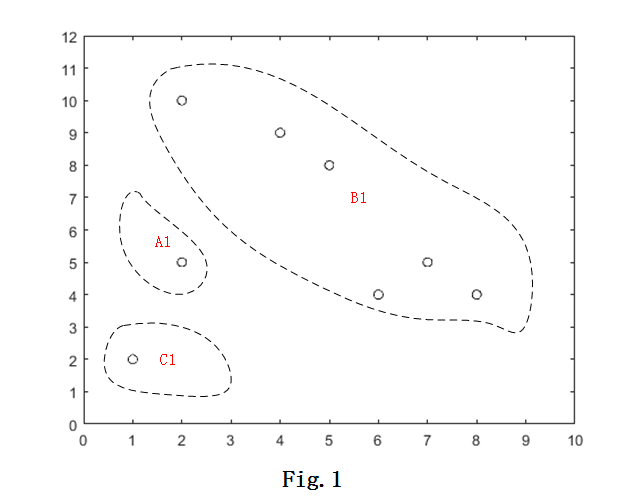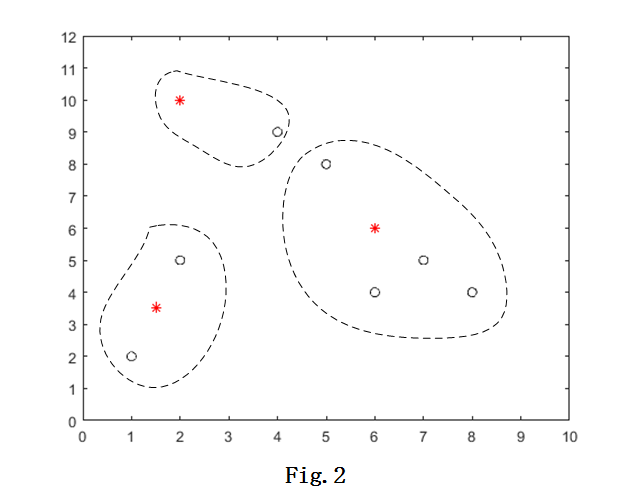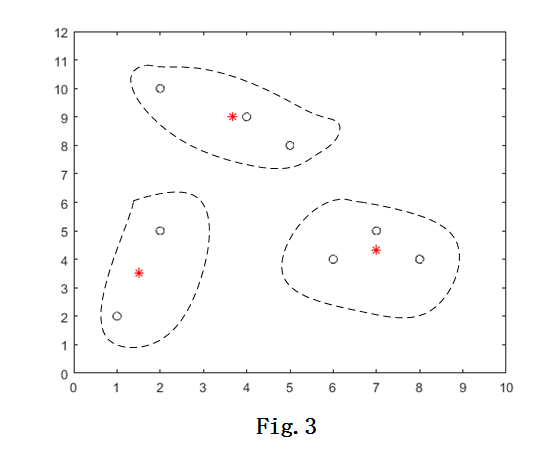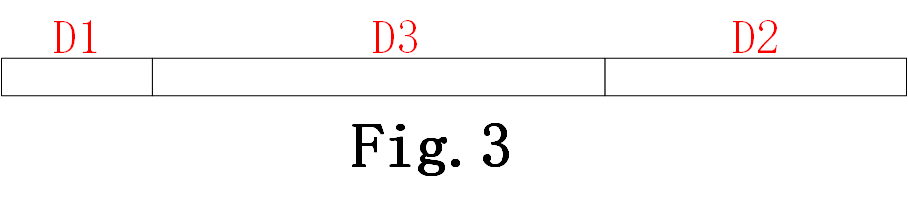聚类分析: k-means算法

k-means算法

1. 假设数据集在一个m$m$维的欧式空间中，我们初始时，可随机选择k$k$个数据项作为这k$k$个簇的形心Ci,i{1,2,k}$C_i, i \in \{1, 2, \dots k\}$，每个簇心代表的其实是一个簇，也就是一组数据项构成的集合。然后对所有的n$n$个数据项，计算这些数据项与Ci$C_i$的距离（一般情况下，在欧式空间中，数据项之间的距离用欧式距离表示）。比如对于数据项Dj,j{1,n}$D_j, j \in \{1, \dots n\}$，它与其中的一个簇心Ci$C_i$最近，则将Dj$D_j$归类为簇Ci$C_i$.
2. 通过上面这一步，我们就初步将D$D$划分为k$k$个类了。现在重新计算这k$k$个类的形心。方法是计算类中所有数据项的各个维度的均值。这样，构成一个新的形心，并且更新这个类的形心。每个类都这样计算一次，更新形心。
3. 对上一步计算得到的新的形心，重复进行第（1），（2）步的工作，直到各个类的形心不再变化为止。1. 时间复杂度：O(nkt)$O(nkt)$，其中，n$n$为数据项个数，k$k$为要聚类成的簇数，t$t$为迭代次数。而通常，k<<n$k<，所以，对于大数据集，k-means算法相对可伸缩，且有效。
2. 局限性：k-means算法有其相应的局限性，我们必须明白这些缺点，才能避免不正确的使用：
• 只能应用于可计算均值的数据，比如对于一些标称属性的数据，就不能使用k-means。所以，后来人们设计了k-众数算法，来解决对于标称属性数据的聚类;
• 必须事先给出要生成的簇数k$k$，而实际上我们大多时候并不知道这些数据应该生成的簇数，后来ISODATA算法通过类的自动合并和分裂，得到较为合理的类型数目k;
• 前面已经说过，k-means对于初始点的选择很重要，不同初始点，会导致不同效果的聚类。为了解决这个问题，k-means++算法应运而生。

k-means算法的在线模式

• 随机选择k$k$个数据点作为簇中心；
• 依次遍历每个数据点，每一次遍历（设当前遍历到的数据点为Di$D_i$）执行下面两步操作：
1. 计算得到距离Di$D_i$最近的簇心，将Di$D_i$分配给这个簇；
2. 立即更新簇心（其实，全部的k$k$个簇中，只有这一个簇需要更新簇心）
3. 循环执行前两步，直到簇心不再改变位置（此时，这个数据点属于哪个簇其实固定了）
• 遍历完所有的数据点，也就完成了聚类；

k-means++算法

k-means++是k-means的变形，通过小心选择初始簇心，来获得较快的收敛速度以及聚类结果的质量，本文中，我们将简单介绍k-means++. 首先，一定要先理解k-means++的原理。1. 对已经选出作为簇心的r$r$个点（r<k$r < k$），计算数据集中每个数据项应该归类的簇，以及距离
2. 将这n$n$个距离求和，得到sum(Dis_i)，然后随机选取一个小于sum(Dis_i)的值Random
3. 令Random依次减去Disi$Dis_i$，Random -= Disi$Dis_i$，直到Random <= 0为止，此时，Random减去的Disi$Dis_i$所对应的数据项就是新的簇心。

1. 随机选择一个数据项，作为第一个簇心
2. 根据选择下一个簇心的操作方法（上面列出的3步），选择下一簇心
3. 重复步骤2，直到得到全部的k$k$个簇心

k-means++虽然在初始簇心的选择上比k-means更优，但是依然也有缺陷，比如，下一个簇心的选择总是依赖于已有的簇心，后来k-means||算法，改进了这一缺点，这里就不再做过多介绍了。

k-means++算法和前面k-means算法的全部代码以及测试数据我都放在了github上：kmeans，欢迎参考指正。